Next: 3.1.4 The Lattice Heat Up: 3.1 Sets of Partial Previous: 3.1.2 The Drift-Diffusion Transport

## 3.1.3 The Hydrodynamic Transport Model

In the hydrodynamic transport model, carrier temperatures are allowed to be different from the lattice temperature. The basic equations (3.1) through (3.3) are augmented by energy balance equations which determine the carrier temperatures. The current relations take the form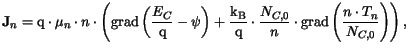(3.6)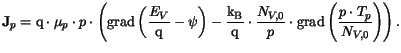(3.7)

The energy balance equations state conservation of the average carrier energies. In terms of the carrier temperatures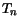and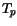they can be written as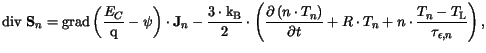(3.8)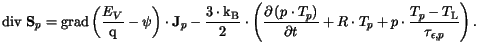(3.9)

Here,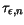and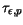denote the energy relaxation times, while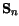and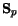are the energy fluxes.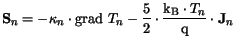(3.10)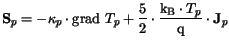(3.11)

The carrier thermal conductivities,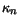and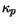, are assumed to obey a generalized Wiedemann-Franz law.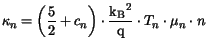(3.12)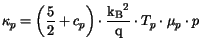(3.13)

The heat capacities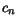and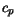are usually neglected (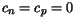).

Strictly speaking, the model described above in this subsection represents an energy transport (ET) model. Such a model is obtained when in the course of deriving the moment equations the average kinetic energy is consequently neglected against the thermal energy, assuming that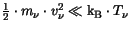. Since in the literature the terms energy transport and hydrodynamic transport are often used synonymously, and since during the development of MINIMOS-NT the term hydrodynamic transport was used, this notation is retained throughout this work.Next: 3.1.4 The Lattice Heat Up: 3.1 Sets of Partial Previous: 3.1.2 The Drift-Diffusion Transport
Vassil Palankovski
2001-02-28